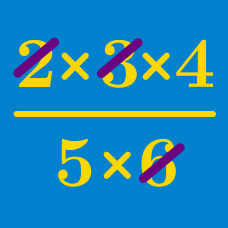Algebra

# Zero Product Property

If $ab = 0$, which of the following statements MUST be true?

If $b \neq c$ and $ab = ac$, which of the following statements must be true?

Solve $x^2-5x=0$.

What are the solutions to $(x-3)(x-5)=0$?

If $a \neq 5$ and

$a x = 5 x ,$

then what is the value of $x$?

×